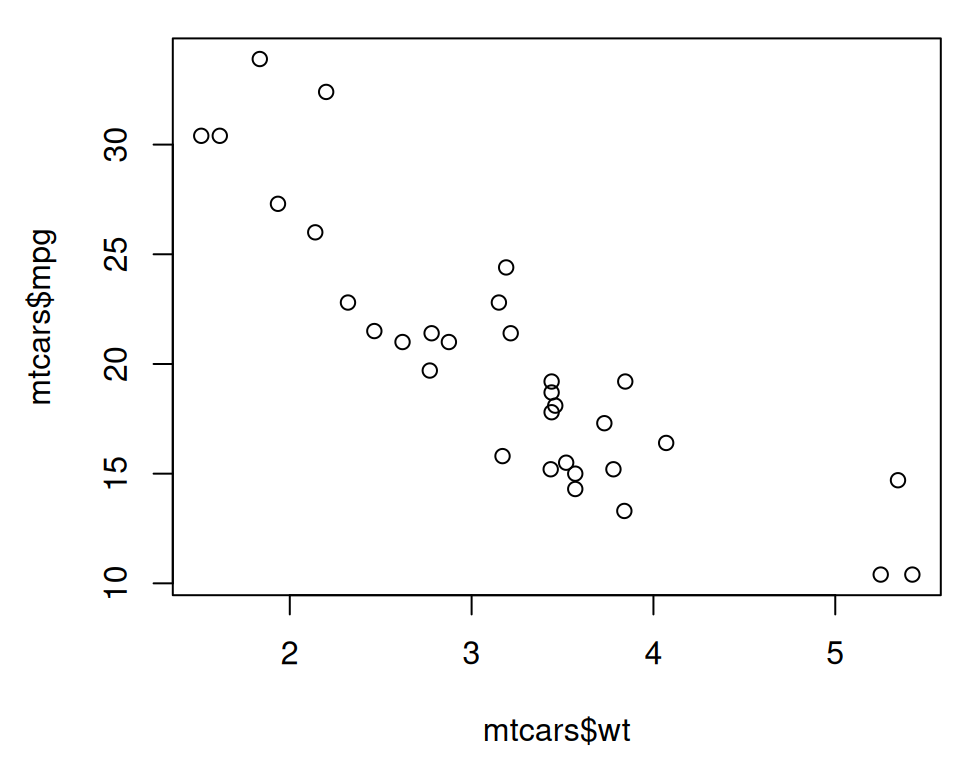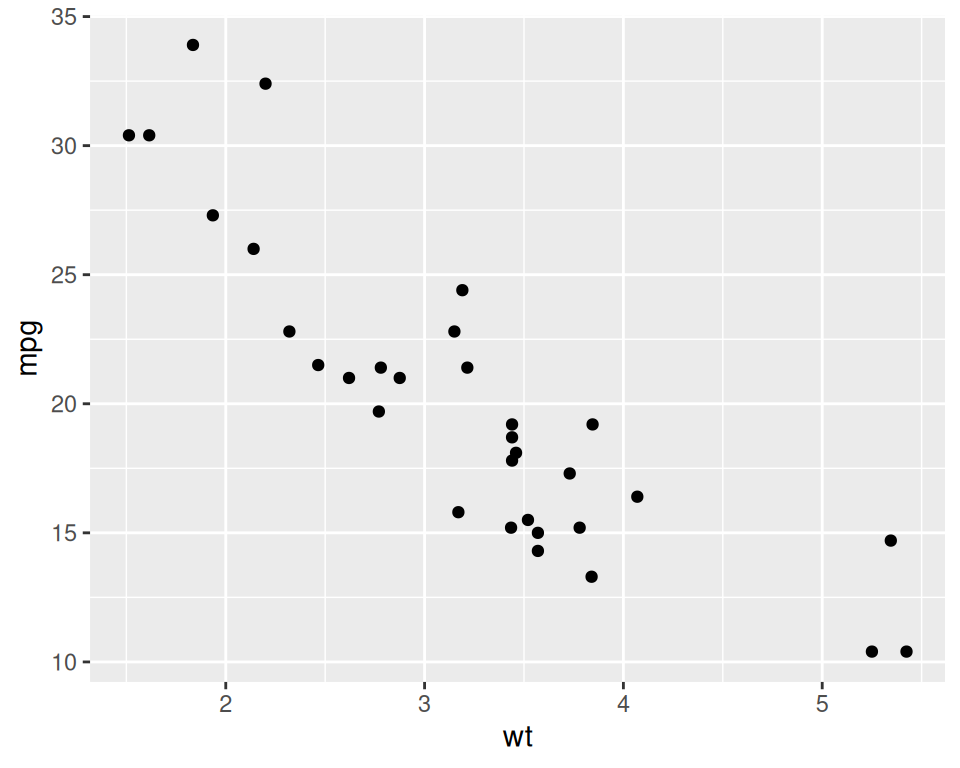## 2.1 Creating a Scatter Plot

### 2.1.1 Problem

You want to create a scatter plot.

### 2.1.2 Solution

To make a scatter plot (Figure 2.1), use `plot()` and pass it a vector of x values followed by a vector of y values:

``plot(mtcars\$wt, mtcars\$mpg)``Figure 2.1: Scatter plot with base graphics

The `mtcars\$wt` returns the column named `wt` from the `mtcars` data frame, and `mtcars\$mpg` is the `mpg` column.

With ggplot2, you can get a similar result using the `ggplot()` function (Figure Figure 2.2):

``````library(ggplot2)

ggplot(mtcars, aes(x = wt, y = mpg)) +
geom_point()``````Figure 2.2: Scatter plot with ggplot2

The first part, `ggplot()`, tell it to create a plot object, and the second part, `geom_point()`, tells it to add a layer of points to the plot.

The usual way to use `ggplot()` is to pass it a data frame (`mtcars`) and then tell it which columns to use for the x and y values. If you want to pass it two vectors for x and y values, you can use `data = NULL`, and then pass it the vectors. Keep in mind that ggplot2 is designed to work with data frames as the data source, not individual vectors, and that using it this way will only allow you to use a limited part of its capabilities.

``````ggplot(data = NULL, aes(x = mtcars\$wt, y = mtcars\$mpg)) +
geom_point()``````

It is common to see `ggplot()` commands spread across multiple lines, so you may see the above code also written like this: